December 15, 2021

# Class 10 Maths Chapter 12 Areas Related To Circles

So far, we have learned quite a bit about circles. We know how to find the area of the circle, the circumference or perimeters of the circle, etc. Not just circles but we know how to find areas and perimeters of other common shapes like triangles, squares, rectangles, parallelograms, etc. We know all these shapes exist in nature, but not exactly as we want. Often we will see things in combination, and this is why class 10 maths chapter 12 areas related to circles is of big importance for practical life.

Although in chapter 10 we learned mostly about tangents, there were some key points that we will need in this chapter also, like, segment, sector, chord, and tangent themselves. So, it’s always better to revest the pre-requisite for a better understanding of a topic.

## Class 10 Maths Chapter 12 Areas Related To Circles

Surely your memory for chapter 10 must still be fresh, but for those of you who don’t remember it very well, here are some of the basic terms that were used in that chapter and will also be used in class 10 maths chapter 12 areas related to circles.

Circumference of the circle: Just like other shapes, the measurement of just the boundary of a circle is called its circumference or perimeter.

Chord of the circle: If we mark two points on the circumference of a circle and connect them with a line segment, then that line segment will be called the chord of that circle.

Diameter & radius: Diameter is simply the longest chord the can be drawn on a circle. If we draw a line segment that joins the center point and any point of the circumference of that circle, then that line segment is called the radius of that circle. Radius is always exactly half of the circle’s diameter

Arc: If we mark two separate points on the circumference of a circle, then the part of the circumference lying between those points is called an arc of that circle. There will always be two arcs on a circle. The bigger one is called the major arc and the small one is called the minor arc.  If both the arcs of a circle are of equal size, in such case both the arcs will be called semicircles.

Segment: The two unequal parts obtained by drawing a chord on a circle are called segments. The part with a bigger area or arc is called a major segment, and the one with a smaller area is called a minor sector of that circle.

Sector: The area between an arc and the two radii is known as a sector. If we draw two separate radii (plural form of radius) of a circle then they will divide the circle into two sectors. The sector with the bigger region is called a major sector, and the one with the smaller region is called a minor sector.

### Class 10 Maths Chapter 12 Areas Related To Circles

We through the basic terminologies that we need to learn class 10 maths chapter 12 areas related to circles. But, this is just the theory, the important part are the formulas that we will need while solving the questions of this chapter. So now, let’s take a quick look at the important formulas for chapter 12.

 Diametre of circle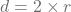, {r → radius, d → diameter} Radius of circle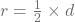{r → radius, d → diameter} Area of circle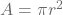Area of triangle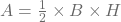{B → base, H → height} Pythagorus theorem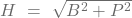Value of θ = 0°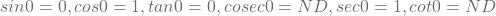Value of θ = 30°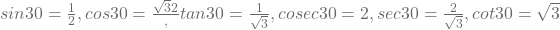Value of θ = 45°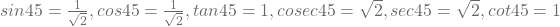Value of θ = 60°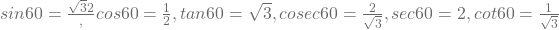Value of θ = 90°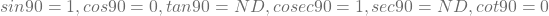Trigonometric ratios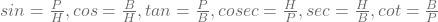{P → perpandicular, B → base, H → height}

You will need all these formulas when you will try to solve the problems of class 10 maths chapter 12 areas related to circles.

#### Class 10 Maths Chapter 12 Areas Related To Circles

With all the basics and formulas fresh in our minds, let’s move on to the main topics of this chapter to solve all the questions from exercises 12.1, 12.2, and 12.3.

# Perimeter and area of a circle

Perimeter or circumference as we have seen earlier in this article is the measure of just the boundary of any shape. And, area, as we know, is the region or part covered by any shape. They are repeating these topics here because they are important to solve the forthcoming problems. There is not much to discuss, so let’s just directly jump to the formulas. The formula for the area of a circle is given above in the table.

Perimeter of circle = 2πr {r is radius of circle}

# Areas of sector and segment of a circle

In class 10 maths chapter 12 areas related to circles, we have already discussed both sector and segment of the circle above in this article. But, we have just read the definition of these two, we don’t know yet how to calculate the areas of these two. So, here are the formulas for both sector and segment.

Area of the sector of angle θ =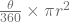Length of an arc of a sector of angle θ =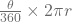Area of segment of a circle = Area of the corresponding sector – Area of the corresponding triangle

# Areas of combinations of plane figures

This part of chapter 12 will teach how one can calculate areas of combined shapes which we mostly see in the real life. e.g how we can obtain the area of the circle inside which a triangle is nested, etc. Again, not much of a theory here, so you will have to directly jump on solving problems and looking at the examples as given in the NCERT book.

Following is the blueprint of class 10 maths chapter 12 areas related to circles:

class 10 maths chapter 12 areas related to circles blueprint
1 Marker 1 question
3 Marker 1 question
Total questions 2
Total marks 4

Blueprint can never be 100% accurate, therefore it’s important to note that questions and their marks as mentioned in the above table may vary in the board exams.